# Texas Go Math Grade 3 Lesson 10.2 Answer Key Number of Equal Groups

Refer to our Texas Go Math Grade 3 Answer Key Pdf to score good marks in the exams. Test yourself by practicing the problems from Texas Go Math Grade 3 Lesson 10.2 Answer Key Number of Equal Groups.

## Texas Go Math Grade 3 Lesson 10.2 Answer Key Number of Equal Groups

Essential Question
How can you model a division problem to find how many equal groups?
By using counters, and putting the same amount into each group then we count each group. The equal groups model used in multiplication can be used to solve division problems as well.
Understand division as an unknown-factor problem.
For example,
find 32 ÷ 8  = 4 by finding the number that makes 32 when multiplied by 8.

When you multiply, you put equal groups together. When you divide, you separate into equal groups.

Unlock the Problem
William has 12 shells and some boxes. He wants to put his shells in groups of 3. How many boxes does he need for his shells?

• Underline what you need to find.
• How many shells does William want in each group? ____
He need to find boxes for his shells.
He need 4 shells in each group
Explanation:
William has 12 shells and some boxes.
He wants to put his shells in groups of 3
Total boxes he need for his shells
12 ÷ 3 = 4

Make equal groups.

• Look at the 12 counters.
• Circle a group of 3 counters.
• Continue circling groups of 3 until all 12 counters are in groups.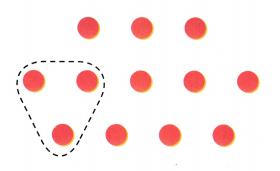There are ___ groups of counters.
So, William needs ___ boxes for his shells.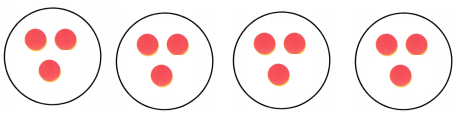There are 4 groups of 3 counters.
So, William needs 4 boxes for his shells.

Math Talk
Mathematical Processes
Explain how the drawing would change if William wanted to put his shells in groups of 4.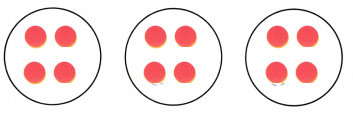Share and Show

Question 1.
Emily has 12 counters. She puts them in groups of 2. Draw a picture to show the number of groups.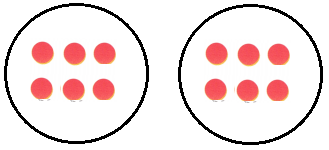Explanation:
Emily has 12 counters.
She puts them in groups of 2.
Total number of groups to show the counters
12 ÷ 2 = 6

Explain how the drawing would change if William wanted to put his shells in groups of 4.

Math Talk
Mathematical Processes
Explain how you find the number of equal groups when you divide.

Draw counters on your Math Board. Then circle equal groups. Complete the table.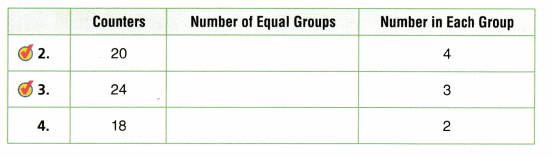Problem Solving

Question 5.
H.O.T. Explain two ways you could divide 18 counters into equal groups. Draw a picture to show each way.
Two ways as follows: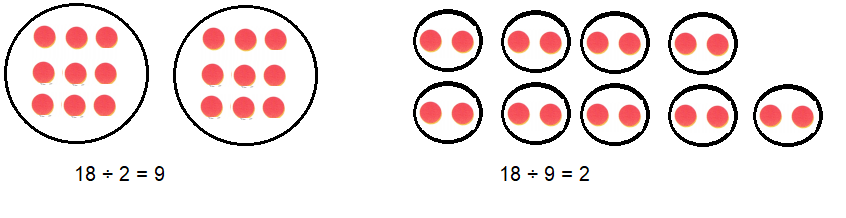Unlock the Problem

Question 6.
A store has 24 beach towels in stacks of 6 towels each. How many stacks of beach towels are at the store?a. What do you need to find?
How many stacks of beach towels are at the store?
4 stacks
24 ÷ 6 = 4

b. How will you use what you know about making equal groups to solve the problem?
By using counters, and putting the same amount into each group then we count each group.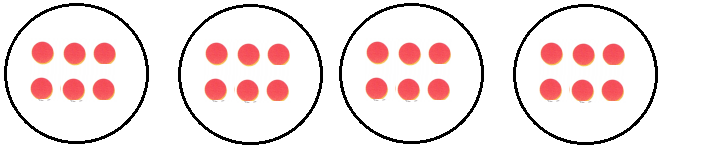Explanation:
each red counter is one beach towel
6 beach towels in one group
4 stacks for 24 beach towel

c. Draw equal groups at the right to find how many stacks of beach towels there are at the store.d. Complete the sentences.

The store has ___ beach towels.
There are ___ towels in each stack.
So, there are ___ stacks of beach towels at the store.
The store has 24 beach towels.
There are 6 towels in each stack.
So, there are 4 stacks of beach towels at the store.

Question 7.
H.O.T. Multi-Step A store has 18 red beach balls and 17 green beach balls in boxes of 5 beach balls each. How many boxes of beach balls are at the store?7 boxes of beach balls.
Explanation:
A store has 18 red beach balls and 17 green beach balls in boxes of 5 beach balls each.
Total beach balls 18 + 17 = 35
Total boxes of beach balls at the store
35 ÷ 5 = 7

Question 8.
H.O.T. Pose a Problem Write a problem about dividing beach toys into equal groups. Solve the problem.
A store has total18 beach toys into equal groups .Each box contains 6 beach toys. How many boxes of beach toys at the store?
Explanation:
A store has total18 beach toys into equal groups
Each box contains 6 beach toys.
Total boxes of beach toys at the store are
18 ÷ 6 = 3

Fill in the bubble for the correct answer choice. You may use models or strategies to solve.

Question 9.
Analyze Jake’s family spends 16 days visiting national parks. They spend 4 days at each national park. How many national parks do they visit?
(A) 4
(B) 12
(C) 64
(D) 20
Option (A)
Explanation:
Analyze Jake’s family spends 16 days visiting national parks.
They spend 4 days at each national park.
Number of national parks they visit?
16 ÷ 4 = 4

Question 10.
Hitomi picks 28 peaches to make pies. She uses 7 peaches in each pie. How many pies does she make?
(A) 21
(B) 3
(C) 35
(D) 4
Option (D)
Explanation:
Hitomi picks 28 peaches to make pies.
She uses 7 peaches in each pie.
Number of pies she make
28 ÷ 7 = 4

Question 11.
Multi-Step Molly is making string bracelets for her friends. She has 25 pieces of blue string and 17 pieces of red string. She uses 7 strings for each bracelet. How many string bracelets can Molly make?(A) 10
(B) 6
(C) 35
(D) 7
Option (B)
Explanation:
Molly has 25 pieces of blue string and 17 pieces of red string.
Total strings she has 25 + 17 = 42
She uses 7 strings for each bracelet.
Total string bracelets Molly make
42 ÷ 7 = 6

Texas Test Prep

Question 12.
Apply Dan’s train is 27 inches long. If each train car is 3 inches long, how many train cars are there?
(A) 9
(B) 6
(C) 7
(D) 8
Option(A)
Explanation:
Apply Dan’s train is 27 inches long.
If each train car is 3 inches long,
Number of train cars
27 ÷ 3 = 9

### Texas Go Math Grade 3 Lesson 10.2 Homework and Practice Answer Key

Draw equal groups to solve. Complete the sentences.

Question 1.
A classroom has 21 desks in rows of 3 desks each. How many rows are there?
The classroom has ___ desks.
There are ___ in each row.
So, there are ___ rows.
The classroom has 21 desks.
There are 3 in each row.
So, there are 7 rows.
Explanation:
A classroom has 21 desks in rows of 3 desks each.
Total rows of desks
21 ÷ 3 =7

Question 2.
The lunchroom has 28 plates in 7 equal stacks. How many plates are in each stack?
The lunchroom has ___ plates.
There are ___ equal stacks.
So, there are ___ plates in each stack.
The lunchroom has 28 plates.
There are 7 equal stacks.
So, there are 4 plates in each stack.
Explanation:
The lunchroom has 28 plates in 7 equal stacks.
Number of plates in each stack
28 ÷ 7 = 4

Problem Solving

Question 3.
There are 35 students in gym class. There are 7 students on each team. How many teams are there?
5 Teams
Explanation:
There are 35 students in gym class.
There are 7 students on each team.
Total teams in gym class
35 ÷ 7 = 5

Question 4.
On a class trip there are 24 students in mini vans. There are 8 students in each van. How many vans are there?
3 vans
Explanation:
On a class trip there are 24 students in mini vans.
There are 8 students in each van.
Total vans required for class trip
24 ÷ 8 = 3

Texas Test Prep

Lesson Check

Question 5.
Lisa has 40 beads to make a ñecklace. She puts the beads in 8 equal groups. How many beads are in each group?
(A) 32
(B) 8
(C) 48
(D) 5
Option (D)
Explanation:
Lisa has 40 beads to make a ñecklace.
She puts the beads in 8 equal groups.
Number of beads in each group
40 ÷ 8 = 5

Question 6.
A store clerk has 18 video games. She puts them in 3 equal groups on some shelves. How many video games are on each shelf?
(A) 15
(B) 6
(C) 21
(D) 5
Option(B)
Explanation:
A store clerk has 18 video games.
She puts them in 3 equal groups on some shelves.
Number of video games on each shelf
18 ÷ 3 = 6

Question 7.
A grocer has 32 bunches of bananas. He puts them in baskets of 8 each. How many baskets does the grocer use?
(A) 40
(B) 24
(C) 6
(D) 4
Option (A)
Explanation:
A grocer has 32 bunches of bananas.
He puts them in baskets of 8 each.
32 ÷ 8 = 4

Question 8.
Jake’s workout includes 25 push-ups. He does them in sets of 5 each. How many sets of push-ups does Jake’s
workout include?
(A) 5
(B) 4
(C) 20
(D) 6
Option (A)
Explanation:
Jake’s workout includes 25 push-ups.
He does them in sets of 5 each.
Total sets of push-ups Jake’s workout include
25 ÷ 5 = 5

Question 9.
Multi-Step Holly has 24 roses and 24 tulips. She wants to put 8 flowers each into a vase. How many vases will Holly need for all of the flowers?
(A) 48
(B) 3
(C) 6
(D) 40
Option (C)
Explanation:
Holly has 24 roses and 24 tulips.
Total flowers 24 + 24 = 48
She wants to put 8 flowers each into a vase.
Number of vases Holly need for all of the flowers
48 ÷ 8 = 6

Question 10.
Multi-Step Roscoe makes a tile design with 18 red tiles and 27 blue tiles. He arranges them in 9 rows. How many tiles are in each row?
(A) 3
(B) 5
(C) 2
(D) 9